PROC TTEST Statement
PROC TTEST <options> ;

The PROC TTEST statement invokes the procedure. Table 95.2 summarizes the options in the PROC TTEST statement by function. The options are then described fully in alphabetical order.

Table 95.2 PROC TTEST Statement Options

Option

Description

Basic Options

Specifies input data set

Determines sort order of CLASS variable or CROSSOVER= treatment variables

Analysis Options

Specifies
confidence level

Specifies distributional assumption (normal or lognormal)

Specifies null value

Specifies number of sides and direction

Specifies test criterion (difference or ratio)

Requests equivalence test and specifies bounds

Displayed Output

Requests confidence interval for standard deviation or CV

Requests Cochrantest

Produces ODS statistical graphics

Output Ordering

Groups results by PAIRED or VAR variables

Groups results by tables

The following options can appear in the PROC TTEST statement.

ALPHA=p

specifies that confidence intervals (except test-based mean confidence intervals when the TOST option is used) are to be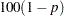% confidence intervals, where
. When the TOST option is used, the test-based mean confidence intervals are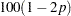% confidence intervals. By default, PROC TTEST uses ALPHA=0.05. Ifis 0 or less, or 1 or more, an error message is printed.

BYVAR

groups the results by the PAIRED or VAR variables. The BYVAR option is enabled by default. Note that this represents a change from previous releases for how the results are grouped with respect to variables and tables. Prior to SAS 9.2, multiple variables were included in each table, similar to the new NOBYVAR option.

CI=EQUAL | UMPU | NONE
CL=EQUAL | UMPU | NONE

specifies whether a confidence interval is displayed for
and, if so, what kind. The CI=EQUAL option specifies an equal-tailed confidence interval, and it is the default. The CI=UMPU option specifies an interval based on the uniformly most powerful unbiased test of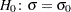. The CI=NONE option requests that no confidence interval be displayed for. The values EQUAL and UMPU together request that both types of confidence intervals be displayed. If the value NONE is specified with one or both of the values EQUAL and UMPU, NONE takes precedence. For more information, see the section Two-Independent-Sample Design.

COCHRAN

requests the Cochran and Cox (1950) approximation of the probability level for the unequal variances situation. For more information, see the section Two-Independent-Sample Design.

DATA=SAS-data-set

names the SAS data set for the procedure to use. By default, PROC TTEST uses the most recently created SAS data set. The input data set can contain summary statistics of the observations instead of the observations themselves. The number, mean, and standard deviation of the observations are required for each BY group (one sample and paired differences) or for each class within each BY group (two samples). For more information about the DATA= option, see the section Input Data Set of Statistics.

DIST=LOGNORMAL | NORMAL

specifies the underlying distribution assumed for the data. The default is NORMAL, unless TEST=RATIO is specified, in which case the default is LOGNORMAL.

H0=m

requests tests against a null value of, unless the TOST option is used, in which caseis merely used to derive the lower and upper equivalence bounds. For the crossover design, the valueapplies for both treatment and period tests. By default, PROC TTEST uses H0=0 when TEST=DIFF (or DIST=NORMAL for a one-sample design) and H0=1 when TEST=RATIO (or DIST=LOGNORMAL for a one-sample design).

NOBYVAR

includes all PAIRED or VAR variables together in each output table. If the NOBYVAR option is not specified, then the BYVAR option is enabled, grouping the results by the PAIRED and VAR variables.

ORDER=DATA | FORMATTED | FREQ | INTERNAL | MIXED

specifies the order in which to sort the levels of the classification variables (which are specified in the CLASS statement) and treatment variables (which are specified in the CROSSOVER= option in the VAR statement). The default is ORDER=MIXED, which corresponds to the ordering in releases previous to SAS 9.2.

This option applies to the levels for all classification or treatment variables, except when you use the ORDER=FORMATTED option with numeric classification or treatment variables that have no explicit format. With this option, the levels of such variables are ordered by their internal value.

The ORDER= option can take the following values:

Table 95.3 continued

Value of ORDER=

Levels Sorted By

DATA

Order of appearance in the input data set.

FORMATTED

External formatted value, except for numeric variables with no explicit format, which are sorted by their unformatted (internal) value.

FREQ

Descending frequency count; levels with the most observations come first in the order. In the event of a tie, ORDER=MIXED is used.

INTERNAL

Unformatted value.

MIXED

Same as ORDER=FORMATTED if the unformatted variable is character-valued; same as ORDER=INTERNAL otherwise (the unformatted variable is numeric-valued).

For FORMATTED and INTERNAL, the sort order is machine-dependent.

For more information about sorting order, see the chapter on the SORT procedure in the Base SAS Procedures Guide and the discussion of BY-group processing in SAS Language Reference: Concepts.

PLOTS <(global-plot-options)> <= plot-request <(options)>>
PLOTS <(global-plot-options)> <= (plot-request <(options)> <... plot-request <(options)>>)>

controls the plots produced through ODS Graphics. When you specify only one plot request, you can omit the parentheses around the plot request. Here are some examples:

```plots=none
plots=(histogram boxplot interval qq profiles agreement)
plots(unpack)=summary
plots(showh0)=interval(type=pergroup)
plots=(summary(unpack) interval(type=period))
```

ODS Graphics must be enabled before requesting plots. For example:

```ods graphics on;

proc ttest plots=all;
var oxygen;
run;

ods graphics off;
```

For more information about enabling and disabling ODS Graphics, see the section Enabling and Disabling ODS Graphics in Chapter 21, Statistical Graphics Using ODS.

If ODS Graphics is enabled but you do not specify the PLOTS option, then PROC TTEST produces a default set of plots. ( Note: The graphical results are unavailable if your input data set contains summary statistics rather than observation values.)

For a one-sample design, the default plots are the following:

• summary plot (histogram with overlaid normal and kernel densities, box plot, and confidence interval band)

• Q-Q plot

For a two-independent-sample design, the default plots are the following:

• summary plot (comparative histograms with overlaid densities and box plots)

• Q-Q plot

For a paired design, the default plots are the following:

• summary plot (histogram, densities, box plot, and confidence interval) of the difference or ratio

• Q-Q plot of the difference or ratio

• profiles plot

• agreement plot

For a crossover design, the default plots are the following:

• comparative histograms with overlaid densities by treatment and period

• comparative box plots by treatment and period

• Q-Q plots by treatment and period

• profiles over treatment plot

• agreement of treatments plot

For more detailed descriptions of plots, see the section Interpreting Graphs.

The global plot options include the following:

ONLY

suppresses the default plots. Only plots specifically requested are displayed.

SHOWH0
SHOWNULL

shows the null value (as specified by the H0= option in the PROC TTEST statement) in all relevant plots. For one-sample and paired designs, the null value can appear in SUMMARY, BOX, and INTERVAL. For two-independent-sample and crossover designs, the null value can appear only in INTERVAL.

UNPACKPANEL
UNPACK

suppresses paneling. By default, multiple plots can appear in some output panels. Specify UNPACKPANEL to get each plot in a separate panel. You can specify PLOTS(UNPACKPANEL) to unpack the default plots. You can also specify UNPACK as a suboption with SUMMARY.

The plot requests include the following:

ALL

produces all appropriate plots. You can specify other options with ALL; for example, to request all plots and specify that intervals should be for the period difference in a crossover design, specify PLOTS=(ALL INTERVAL(TYPE=PERIOD)).

AGREEMENT
AGREEMENTPLOT

requests an agreement plot. This plot is produced by default for paired and crossover designs, the only designs for which the AGREEMENT option is valid.

For paired designs, the second response in each pair is plotted against the first response. See the section Agreement Plots for Paired Designs for further details.

For crossover designs, the AGREEMENT plot request has the following options:

TYPE=PERIOD

plots the response in the second period against the response in the first period. See the section Period Agreement Plots for Crossover Designs for further details.

TYPE=TREATMENT

plots the response associated with the second treatment against the response associated with the first treatment. This is the default TYPE= option for crossover designs. See the section Treatment Agreement Plots for Crossover Designs for further details.

BOX
BOXPLOT

requests a box plot or comparative box plots. This plot is produced by default for crossover designs. For other designs, a box plot appears as part of the SUMMARY plot by default.

For one-sample and paired designs, a confidence interval for the mean is shown as a band in the background, along with the equivalence bounds if the TOST option is used in the PROC TTEST statement.

For a two-independent-sample design, comparative box plots (one for each class) are shown. For a crossover design, comparative box plots for all four combinations of the two treatments and two periods are shown.

See the section Box Plots for further details.

HISTOGRAM
HIST
HISTDENS

requests a histogram or comparative histograms with overlaid normal and kernel densities. This plot is produced by default for crossover designs. For other designs, it appears as part of the SUMMARY plot by default.

For one-sample and paired designs, the histogram and densities are based on the test criterion (which is the mean difference or ratio for a paired design). For a two-independent-sample design, comparative histograms (one for each class) are shown. For a crossover design, histograms for all four combinations of the two treatments and two periods are shown.

See the section Histograms for further details.

INTERVAL
INTERVALPLOT

requests plots of confidence interval for means.

For a two-independent-sample design, the INTERVAL plot request has the following options:

TYPE=PERGROUP

shows two separate two-sided confidence intervals, one for each class. This option cannot be used along with the SHOWH0 global plot option.

TYPE=TEST

shows pooled and Satterthwaite confidence intervals. This is the default TYPE= option for two-independent-sample designs.

For a crossover design, The INTERVAL plot request has the following options:

TYPE=PERGROUP

shows four separate two-sided intervals, one for each treatment-by-period combination. This option cannot be used along with the SHOWH0 global plot option.

TYPE=PERIOD

shows pooled and Satterthwaite confidence intervals for the period difference or ratio. This option is invalid if the IGNOREPERIOD option is used in the VAR statement.

TYPE=TREATMENT

shows pooled and Satterthwaite confidence intervals for the treatment difference or ratio. This is the default TYPE= option for crossover designs.

See the section Confidence Intervals for further details.

NONE

suppresses all plots.

PROFILES
PROFILESPLOT

requests a profiles plot. This plot is produced by default for paired and crossover designs, the only designs for which the PROFILES option is valid.

For paired designs, a line is drawn for each observation from left to right connecting the first response to the second response. See the section Profiles for Paired Designs for further details.

For crossover designs, the PROFILES plot request has the following options:

TYPE=PERIOD

shows response profiles over period, connecting the first period on the left to the second period on the right for each subject. See the section Profiles over Period for Crossover Designs for further details.

TYPE=TREATMENT

shows response profiles over treatment values, connecting the first treatment on the left to the second treatment on the right for each observation. This is the default TYPE= option for crossover designs. See the section Profiles over Treatment for Crossover Designs for further details.

QQ
QQPLOT

requests a normal quantile-quantile (Q-Q) plot. This plot is produced by default for all designs.

For two-sample designs, separate plots are shown for each class in a single panel. For crossover design, separate plots are shown for each treatment-by-period combination in a single panel.

See the section Q-Q Plots for further details.

SUMMARY
SUMMARYPLOT

requests HISTOGRAM and BOX plots together in a single panel, sharing common X axes. This plot is produced by default for one-sample, paired, and two-independent-sample designs, the only designs for which the SUMMARY option is valid. See the documentation for the BOX and HISTOGRAM plot requests for details. The SUMMARY plot request has the following option:

UNPACK

plots histograms with overlaid densities in one panel and box plots (along with confidence interval bands, if one-sample or paired design) in another. Note that specifying PLOTS(ONLY)=SUMMARY(UNPACK) is exactly the same as specifying PLOTS(ONLY)=(BOX HISTOGRAM).

SIDES=2 | L | U
SIDED=2 | L | U
SIDE=2 | L | U

specifies the number of sides (or tails) and direction of the statistical tests and test-based confidence intervals. The values are interpreted as follows:

SIDES=2

(the default) specifies two-sided tests and confidence intervals for means.

SIDES=L

specifies lower one-sided tests, in which the alternative hypothesis indicates a mean less than the null value, and lower one-sided confidence intervals between minus infinity and the upper confidence limit.

SIDES=U

specifies upper one-sided tests, in which the alternative hypothesis indicates a mean greater than the null value, and upper one-sided confidence intervals between the lower confidence limit and infinity.

TEST=DIFF | RATIO

specifies the test criterion. Use TEST=DIFF to test the difference of means and TEST=RATIO to test the ratio of means. The default is DIFF, unless DIST=LOGNORMAL is specified, in which case the default is RATIO. This option is ignored for one-sample designs.

TOST ( <lower ,> upper )

requests Schuirman’s TOST equivalence test. The upper equivalence bound must be specified. If TEST=DIFF, then the default value for the lower equivalence bound is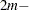upper, whereis the value of the H0= option. If TEST=RATIO, then the default value for lower is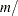upper.